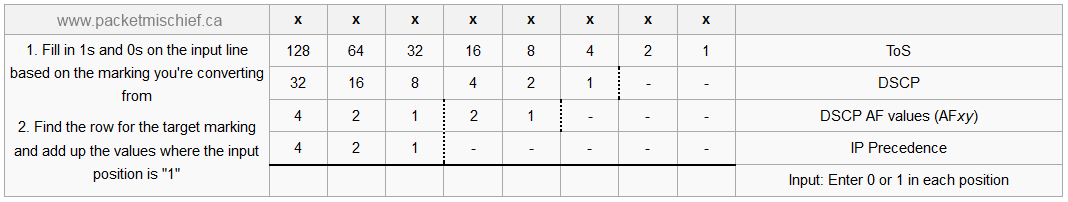This is a quick calculator I came up that I could use in the CCIE lab to translate between various IPv4 header QoS markings. As long as I could remember how to draw out the calculator, all I had to do was some basic math and I could translate between markings quite easily.

This post does not explain what the header fields are, why there's so many or what the significance of one QoS value is over another. I'm making an assumption that the reader is already familiar with QoS concepts.

Here's the calculator:

x x x x x x x x
128 64 32 16 8 4 2 1 ToS
32 16 8 4 2 1 - - DSCP
4 2 1 2 1 - - - DSCP AF values (AF*xy*)
4 2 1 - - - - - IP Precedence
Enter 0 or 1 in each position

Each column represents one bit from the Type of Service (ToS) field in the IPv4 header. The right-most column describes what each row is for. The very last row is meant to be filled in by us; it's the input into the calculator.

As an example, let's say we had a DSCP marking with a decimal value of 46. This is our input into the calculator. We will focus on the "DSCP" row and the input row:

 32 16 8 4 2 1 - - DSCP Enter 0 or 1 in each position

Note that since the DSCP field in the IP header is only 6 bits, there's only 6 bit positions available in this row of the calculator.

Now we fill in our input value of 46 into the calculator. To get 46 we need to turn "on" positions 32, 8, 4, and 2 by placing a "1" in that position on the input row and a "0" in the rest:

 32 16 8 4 2 1 - - DSCP 1 1 1 1 0 Enter 0 or 1 in each position

Now let's say we want to convert this to a ToS value (which is useful when doing an extended ping in IOS as the ping command will take the ToS value as a decimal number). We focus our attention now on the marking that we want to convert to, in this case the ToS:

 128 64 32 16 8 4 2 1 ToS 1 1 1 1 Enter 0 or 1 in each position

The values on the input row are the values I entered above for DSCP 46; those don't change. Now add together every position that is turned on by a "1" to get a ToS of 184 (128+32+16+8). What we've calculated is that a DSCP value of decimal 46 is equal to a ToS value of decimal 184.

Let's do another one, this time from ToS 104 to AFxy. On the AF line of the calculator, there's a dashed vertical line between the 3rd and 4th bit positions. This denotes the boundary between the bit fields that make up the x value and the y value.

 128 64 32 16 8 4 2 1 ToS 4 2 1 2 1 - - - DSCP AF values (AF*xy*) 1 1 1 Enter 0 or 1 in each position

I've entered the input values that correspond to ToS 104 (64+32+8). Now do the conversion to AF and you get x = 3 (2+1) and y = 1, therefore ToS 104 equates to AF31.

Here's a PNG image of the calculator for reference (click to enlarge).Happy calculating.

Disclaimer: The opinions and information expressed in this blog article are my own and not necessarily those of Cisco Systems.